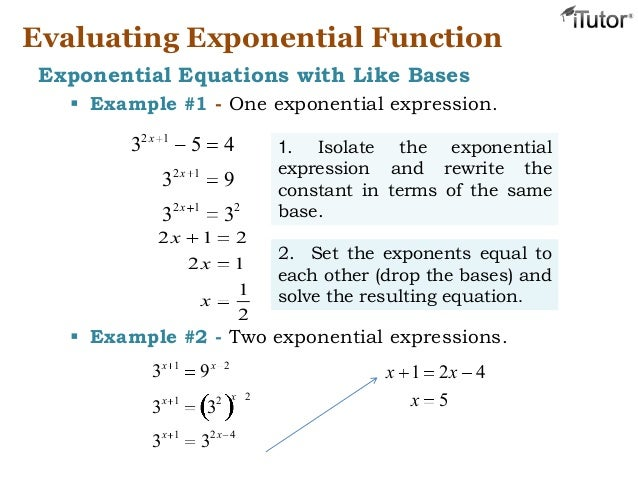# Linear and Exponential Functions & thier Graphs

lesson 8-8 exponential growth and decay

Exponent_Rules_Practice

modelling exponential functions

exp

Exponential_Growth_Worksheet

Exp_Growth_Practice2

Exponential growth and decay worksheet

Practice Test year 11 Growth and Decay

Exponential Functions (powerpoint slides)# Exponential Equations: Exponential Growth and Decay Application

A common application of exponential equations is to model exponential growth and decay such as in populations, radioactivity and drug concentration.

The formula for exponential growth and decay is:Exponential Decay Goals: Understand the nature of exponential decay Write exponential model given initial value and decay factor or decay rate Understand the difference between decay factor and decay rate Terms to know: ·         decay ·         decay factor ·         decay rate ·         exponential function Example 1 Suppose now we were considering the population of a certain community.  Suppose also that 120,000 people lived there in 2005 and that 2% of the population leave every year.   How many people would be living there in 2008? After the first year, the population =   Original population  –  2% of original population =   120,000 – .02(120,000) =   120,000 – 2400 =   117,600. Note that 2400 people left town during the first year. After the second year, the population =   Former population – 2% of former population =   117,600 – .02(117,600) =   117,600 – 2352 =   115,248. Note that 2352 people left town during the second year, which is less than the number that left during the first year. After the third year, the population = Former population – 2% of former population = 115,248 – .02(115,248) = 115,248  2304.96 112,943 (rounding to the nearest person) Note that 2305 people left town during the third year, which is less than the number that left during the second year. Example 2 Now, to generalize as we did for the salary example,

 After the first year, the population is Factor out the GCF, 120,000: After the second year, the population is Factor out the GCF, 120,000(.98): After the third year, the population is Factor out the GCF, 120,000(.98)2: If we let t  = number of years since 2005, and continue in the above fashion, we find that the population, P, depends upon t: Population(t) =

 A function of the form A(t) = Cat  where a > 0 and a  1  is an exponential function.   The number C gives the initial value of the function (when t = 0) and the number a is the growth (or decay) factor.   If a  >  1, the function represents growth; If  0  <   a   <   1, the function represents decay. Note that if the decay rate is r,  the decay factor is 1 – r

 Checkpoint Exponential Decay 1 Example 3.    Identify each of the following as a growth or decay exponential function.  Identify the growth or decay factor, the growth or decay rate, and the initial value.

 a) d) b) e) c) f)

SOLUTION

 a) This function represents growth since the factor is greater than 1. The  growth factor is 1.03.  The growth rate is 3% The initial value is 100. b) This function represents decay since the factor is less than 1. The  decay factor is .  The decay rate is 25%. The initial value is 20 c) This function represents growth since the factor is greater than 1. The  growth factor is 3.  The growth rate is 200%. The initial value is 0.1 d) This function represents decay since the factor is less than 1. The  decay factor is 0.4.  The decay rate is 60%. The initial value is 1. e) We can rewrite the expression on the right using the definition of negative exponents: This function represents decay since the factor is less than 1. The  decay factor is .  The decay rate is 80%. The initial value is 10. f) This one is tricky.  The factor is bigger than one, so one would assume that this function  represents growth.  The negative sign out front, however, means that all values are getting bigger in the negative direction. We say this function represents negative growth with a factor of 5.  The rate is 400%. The initial value is -2